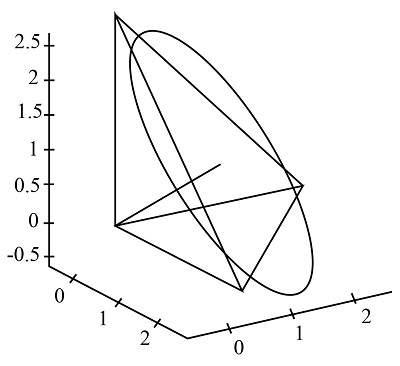# Draw the circle of radius 2 centered at (1,1,1) and lying on the plane x + y + z = 3....

## Question:

Draw the circle of radius {eq}2 {/eq} centered at {eq}\left(1,1,1\right) {/eq} and lying on the plane {eq}x + y + z = 3 {/eq}.

Parameterize the circle. (Hint: Find two orthogonal unit vectors which are parallel to the plane.)

## Parametric Equation

When both the variables of a function get substituted by an unknown variable (parameter), then the whole equation is converted in the form of a parametric equation. The parameters used to substitute these variables are commonly t and {eq}\theta{/eq}.

Given:

Center at {eq}\left( {1,1,1} \right) {/eq}

Plane {eq}= x + y + z = 3 {/eq}

To find:

Draw the Circle.

Parametrize the circle.As shown in the figure, plane {eq}x + y + z = 3 {/eq} is given by points vertices,

{eq}\left( {3,0,0} \right),\left( {0,3,0} \right),\left( {0,0,3} \right). {/eq}

Normal vector to the plane is,

{eq}U = \left( {1,1,1} \right) {/eq}

Let's take another arbitrary vector, V which is orthogonal to U.

A vector is said to orthogonal to another vector when the dot product of both vectors is 0.

Arbitrary vector {eq}V = \left( {1, - 1,0} \right) {/eq} with magnitude {eq}\sqrt 2 {/eq}

Consider a vector W = which is orthogonal to both U and V,

Hence the cross product is,

{eq}\begin{align*} W &= U \times V\\ W &= \left( {1,1,1} \right) \times \left( {1, - 1,0} \right)\\ W &= \left( {1,1, - 2} \right) \end{align*} {/eq}

Magnitude of vector W is {eq}\sqrt 6 . {/eq}

The circle is characterized by the set of the points M,

Such that,

{eq}M = C + 2\left( {\cos \left( t \right)\left( {\dfrac{1}{{\sqrt 2 }}} \right)V + \sin \left( t \right)\left( {\dfrac{1}{{\sqrt 6 }}} \right)W} \right) {/eq}

Where C denotes the center.

And, 2 denotes the radius of the circle.

Substitute the value of C and value of vector V and W in M for finding the value of coordinates.

Coordinates:

{eq}\begin{align*} x &= 1 + 2\cos \left( t \right)\left( {\dfrac{1}{{\sqrt 2 }}} \right) + 2\sin \left( t \right)\left( {\dfrac{1}{{\sqrt 6 }}} \right)\\ y &= 1 - 2\cos \left( t \right)\left( {\dfrac{1}{{\sqrt 2 }}} \right) + 2\sin \left( t \right)\left( {\dfrac{1}{{\sqrt 6 }}} \right)\\ z &= 1 - 4\sin \left( t \right)\left( {\dfrac{1}{{\sqrt 6 }}} \right) \end{align*} {/eq}

Hence,

The circle is Parametrize as follows,

{eq}\begin{align*} x &= 1 + 2\cos \left( t \right)\left( {\dfrac{1}{{\sqrt 2 }}} \right) + 2\sin \left( t \right)\left( {\dfrac{1}{{\sqrt 6 }}} \right)\\ y &= 1 - 2\cos \left( t \right)\left( {\dfrac{1}{{\sqrt 2 }}} \right) + 2\sin \left( t \right)\left( {\dfrac{1}{{\sqrt 6 }}} \right)\\ z &= 1 - 4\sin \left( t \right)\left( {\dfrac{1}{{\sqrt 6 }}} \right) \end{align*} {/eq}